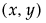The Fraction of Design Space Plot shows the proportion of the design space over which the relative prediction variance lies below a given value. Fraction of Design Space Plot shows the Fraction of Design Space plot for the Bounce Data.jmp sample data table, located in the Design Experiment folder.
Fraction of Design Space Plot
The X axis in the plot represents the proportion of the design space, ranging from 0 to 100%. The Y axis represents relative prediction variance values. For a pointthat falls on the blue curve, the value x is the proportion of design space with variance less than or equal to y. Red dotted crosshairs mark the value that bounds the relative prediction variance for 50% of design space.
Fraction of Design Space Plot shows that the minimum relative prediction variance is slightly less than 0.3, while the maximum is below 1.4. (The actual maximum is 1.395833, as shown in Prediction Variance Profile Showing Maximum Variance.) The red dotted crosshairs indicate that the relative prediction variance is less than 0.34 over about 50% of the design space. You can use the crosshairs tool to find the maximum relative prediction variance that corresponds to any Fraction of Space value. Use the crosshairs tool in Fraction of Design Space Plot to see that 90% of the prediction variance values are below approximately 0.55.

Help created on 9/19/2017